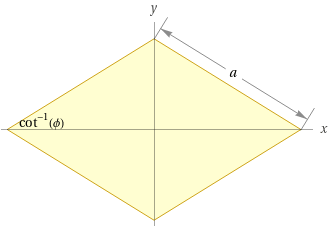Search IntMath
CloseQuadrilaterals are two-dimensional polygons, or shapes with four sides and four angles. They are the most common type of polygon, and can be found in many everyday objects. In geometry, quadrilaterals are studied in great detail.

## Parallelograms

A parallelogram is a quadrilateral with two pairs of parallel sides. The opposite sides of a parallelogram are equal in length, and the opposite angles are also equal. The area of a parallelogram is equal to the product of its base and height.

An important property of a parallelogram is that the line joining the midpoints of the sides is parallel to the opposite sides. This can be used to prove that the diagonals of a parallelogram bisect each other.

## Rectangles

A rectangle is a parallelogram with four right angles. The opposite sides of a rectangle are equal in length, and the diagonals bisect each other and are equal in length. The area of a rectangle is equal to the product of its length and width.

Another important property of a rectangle is that the sum of the angles is 360 degrees. This can be used to prove that the diagonals of a rectangle are equal in length.

## Squares

A square is a special type of rectangle in which all four sides are equal in length. The diagonals of a square are equal in length, and bisect each other at right angles. The area of a square is equal to the square of its side.

An important property of a square is that the angles are all right angles. This can be used to prove that the diagonals of a square are equal in length.

## Practice Problems

1. Find the area of a rectangle with a length of 5 cm and a width of 3 cm.

Answer: The area of the rectangle is 15 cm2.

2. Find the area of a parallelogram with a base of 6 cm and a height of 4 cm.

Answer: The area of the parallelogram is 24 cm2.

3. Find the length of the diagonal of a square with a side of 4 cm.

Answer: The length of the diagonal of the square is 5.66 cm.

4. Find the length of the diagonal of a rectangle with sides of 5 cm and 3 cm.

Answer: The length of the diagonal of the rectangle is 5.83 cm.

5. Find the area of a square with a side of 5 cm.

Answer: The area of the square is 25 cm2.

## Summary

In this article, we discussed the basics of quadrilaterals in geometry. We discussed the properties of parallelograms, rectangles, and squares. We also provided some practice problems to help you understand the concepts. Quadrilaterals are an important part of geometry, and it is important to understand their properties.

## FAQ

### How do you explain quadrilaterals?

A quadrilateral is a four-sided polygon with four angles. Quadrilaterals are classified according to their properties, such as the lengths of their sides and the measures of their angles. They include squares, rectangles, rhombuses, trapezoids, parallelograms, and kites.

### What is a quadrilateral and examples?

A quadrilateral is a four-sided polygon with four angles. Examples of quadrilaterals include squares, rectangles, rhombuses, trapezoids, parallelograms, and kites.

### What are the 7 types of quadrilaterals?

The seven types of quadrilaterals are squares, rectangles, rhombuses, trapezoids, parallelograms, kites, and quadrilaterals with no particular name (also known as irregular quadrilaterals).

### What are the 4 properties of a quadrilateral?

The four properties of a quadrilateral are the lengths of its sides, the measures of its angles, the area, and the perimeter. These properties can be used to classify quadrilaterals into different types.

## Problem SolverThis tool combines the power of mathematical computation engine that excels at solving mathematical formulas with the power of GPT large language models to parse and generate natural language. This creates math problem solver thats more accurate than ChatGPT, more flexible than a calculator, and faster answers than a human tutor. Learn More.